# TOPEX/Poseidon Satellites

Aqua

Aquarius

Jason-1

QuikSCAT

SeaWiFS

Terra’s
MODIS

TOPEX/
Poseidon

## How does an altimeter system work?

To measure sea surface height, it is necessary to know two things: the precise distance between the satellite and the center of the Earth (D1), and the precise distance between the satellite and the sea surface (D2). The height of the sea surface above the center of the Earth is calculated by subtracting D2 from D1.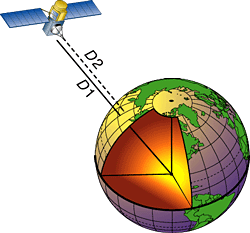The distance between the satellite and the center of the Earth is obtained by knowing the position of the satellite. This is determined using laser tracking ground stations, the Global Position System (GPS) [see below], and other systems such as DORIS. The radar altimeter calculates the distance between the satellite and the sea surface by measuring the time it takes for a pulse of microwave energy transmitted from the satellite to reach the sea surface, reflect off it, and return to the satellite. The pulse travels at a speed of roughly 300,000 km/sec (187,000 miles/sec). The distance between the satellite and the sea surface can be estimated simply by using this speed and the following relationship:

Distance = speed multiplied by time/2

The time is divided by 2 to account for the two-way trip to the sea surface and back.

The time for the round trip is about 0.005 seconds, and it can be measured accurately enough with highly sophisticated clocks to calculate the height of the sea surface to within a few centimeters. The result of the above measurements and calculations yields the height of the sea surface above the center of the Earth. Scientists look at how this height changes every time the satellite passes over the same spot. These changes are called sea surface height anomalies.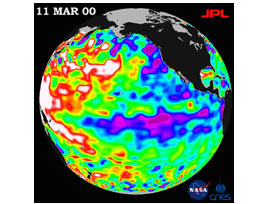Sea level anomalies in millimeters compared to a mean sea level. Taken from: Ocean Surface Topography from Space

By monitoring sea surface height, scientists are able to estimate the speed and direction of ocean currents much like atmospheric scientists use atmospheric pressure variations to determine wind speed and direction. Knowing the motion of ocean currents enables oceanographers to relate them to the wind patterns. Altimetry data can also be related to sea surface temperature and to heat flux at the air-sea interface, and ultimately they can be used to develop more accurate climate models. Most importantly, monitoring the entire oceans using satellites should ultimately help us to determine the impact of humans on the climate of our planet.

## What is The Global Positioning System (GPS)?

The Global Positioning System (GPS) is a worldwide radio-navigation system formed from a constellation of 24 satellites and their ground stations.## How does GPS work?

A GPS receiver measures the distance to a satellite using the travel time of radio signals. By accurately measuring the distance from 3 satellites it is possible to find your position anywhere on Earth. For GPS to work we must be able to 1) measure travel time very accurately, 2) know the exact location of a satellite in space, and 3) understand the delays the signal experiences as it travels through the atmosphere.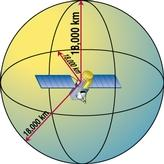Suppose we measure our distance from a satellite and find it to be 18,000 km (11,000 miles). Knowing that we are 18,000 km from a particular satellite narrows down our possible locations to the surface of a sphere centered on the satellite with a radius of 18,000 km as shown in the figure.

If we measure our distance from a second satellite, we now know that we are somewhere on a second sphere centered on the second satellite. We can thus narrow our location to be on the circle where the two sphere's intersect. This is the same thing as when you blow bubbles and two of the bubbles come together. There connection is along a circle.

## Two spheres intersect in a circle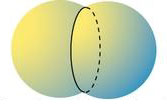## Three spheres intersect in two points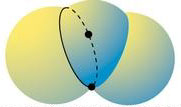If we measure our distance from a third satellite, we know that our location is one of two points where the three spheres intersect. The intersection of three spheres is illustrated in the figure. We will be able to reject one of the points because it will be off the Earth's surface; the other point is our location on Earth.

## Measuring travel time and converting it to distance

The distance to a satellite is determined by measuring how long a radio signal takes to reach the receiver from the satellite. A radio signal travels at the speed of light or roughly 300,000 km/sec (187,000 miles/sec).

Multiply travel time by the speed of light to get distance.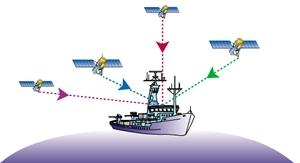GPS satellites have atomic-accuracy clocks and so their timing is very accurate. GPS receivers do not have these clocks, otherwise they would be too expensive to buy and use. Receiver clocks don't have to be too accurate though, because an extra satellite distance measurement can be used to remove timing errors. Therefore, although in theory only 3 satellites are needed to locate yourself, in practice 4 satellites are used to reduce errors in measuring travel time. The figure shows the ship receiving signals from 4 GPS satellites.

Text from Women Exploring the Oceans.